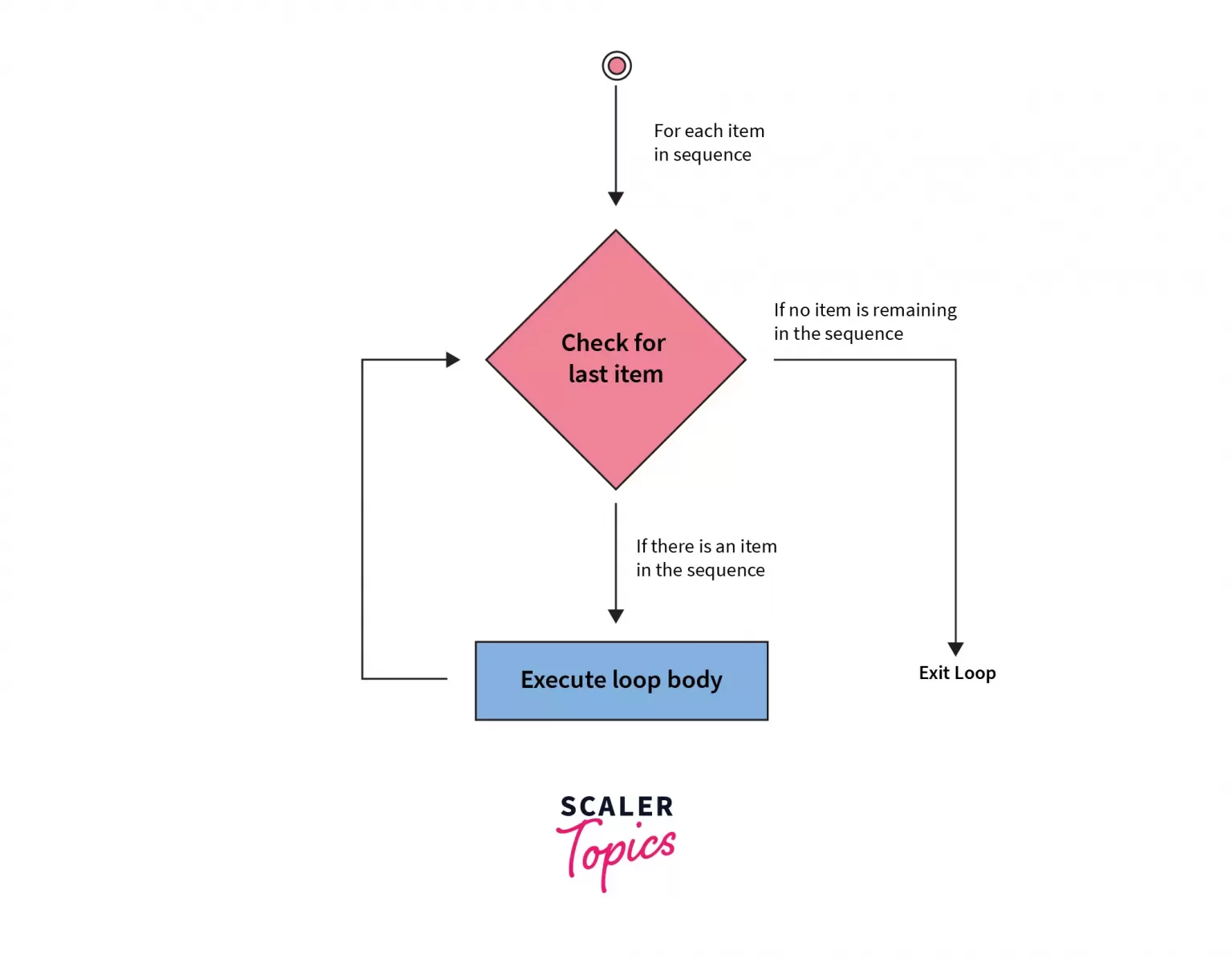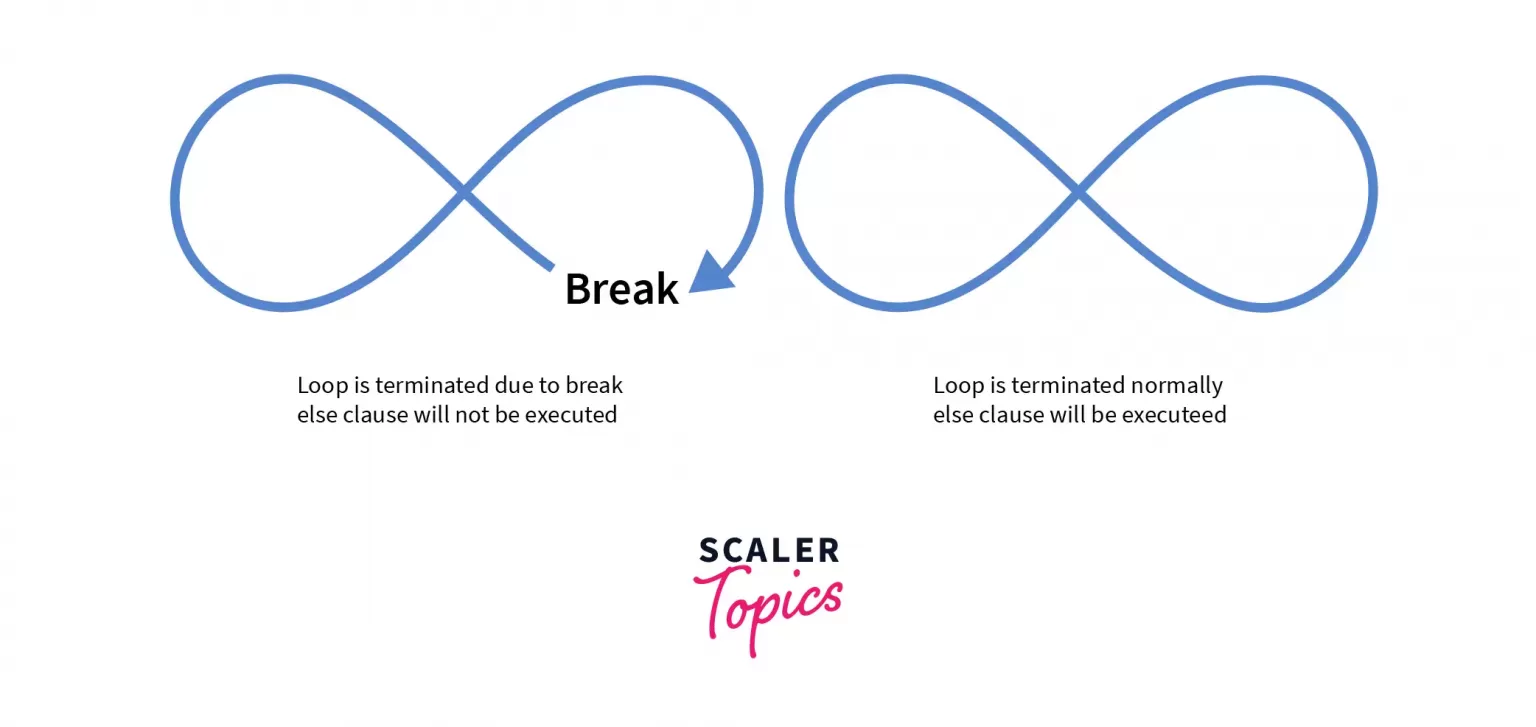# Python For Loops

Challenge Inside! : Find out where you stand! Try quiz, solve problems & win rewards!
Video TutorialIterating over a sequence using a for loop in Python (that is, a list, a tuple, a dictionary, a set, or a string). This functions more like an iterator method found in object-oriented programming languages than the for loop in other programming languages.

Let’s see some Python programs that iterate over a list using a for loop.

Example 1:

Output:

Example 2:

Output:

## for Loop Syntax

Above val is an iterator variable that takes the value of each item from the sequence on each iteration. After taking the value in val, the loop body executes. for loop continues until the last item of the sequence is reached.

## Flowchart of for Loop in Python## Looping through a String

In Python, we can loop through a string. The string is an iterable object containing a sequence of characters. Although Strings are immutable, we can perform operations using the characters to get the desired result.

Let's see the above methods in the below code snippet. There are many ways of iterating through the string in Python using a for loop.

• Method 1: We can iterate over the string character by character until the string gets exhausted. The for loop in each iteration takes one letter from the string, stores it in the variable character, and then prints it.
• Method 2: Another way to iterate over the string is using indexing. Since indexing starts at 0, we can use the range() function, which we have seen above. The range() function will take the stop value as an argument, which serves as the end value for the loop.
• Method 3: One more way to iterate over the string is the enumerate() method. The enumerate() takes the string as an argument and returns a key-value pair. The key is the index number by default and the value of the corresponding letter in the string. The key can also be other numbers as a counter, the starting of which can be passed as an argument.

Output:

## The break Statement

The break is a loop control statement. Using the break statement, we can stop the loop or take out the execution control from the loop before its completion. We stop the further execution of the for loop usually based on some condition(s). Whenever the specified condition is encountered, we use a break statement that takes us out of the Python for a loop. However, the break statement can be used without the condition. But with the for loop, it is usually accompanied by condition(s) based on the logic of our program when we want to come out from the loop.

Below is the code snippet that shows how it works. The Python for loop iterates through a list of integers. In each iteration, an integer is taken into the variable ‘i’ and checked if it is even or not. If the number stored in i is even, then the break statement is executed, and the control comes out of the loop else the odd numbers are printed.

Output:

## The continue Statement

The continue is a loop control statement. Using the continue statement, we can stop the current iteration of the loop based on some condition(s), and control again comes to the beginning of the loop with the next iteration. Similar to the break statement, a continue statement does not necessarily have to be accompanied by a condition. The continue statement can be used without condition. But generally, within the for loop in Python, we find its condition application to skip some statements in certain iterations.

Let’s see a Python code example below to understand it better. In this, we iterate over a list of different country names and print them. But we want that if the name ‘China’ is encountered, then the name is not printed. For this purpose, we have a condition accompanied by a continue statement that skips the print command and initiates the subsequent iteration of the loop.

Output:

In the above code, you can see that when the value of x is equal to China, the loop doesn’t execute fully, and the control goes back to the next iteration. So, this was just a simple case of using a continue statement in Python for loops. We can have many other applications of it depending on the program we are making.

## The range() Function

range() is a built-in function provided by Python. This function is commonly used with a for loop for looping over a range of numbers. This function returns a sequence of numbers that, by default, starts with zero, increments by 1, and ends at a specified number passed as an argument. However, it is possible to provide this function as an argument for the starting number, increment step size, and the number at which it needs to stop. There are three ways in which a range() function can be used:

• range(stop): It takes one argument and is the default version of the range() function. It will produce a sequence of numbers starting from 0, incrementing by 1, and ending at the specified number count. For example, range(15) will generate the numbers starting at 0 and ending at 14.
• range(start, stop): This will take as an argument the starting and the ending numbers and will generate the sequence incrementing by 1. For example, range(5, 10) will generate a sequence of numbers starting at 5 and ending at 9, by default incremented by 1.
• range(start, stop, step/Increment): This version takes the start value, step value by which the series will increment(positive integer) or decrement(negative integer), and ending values parameters and generates the sequence. For example, range(6, 21, 2) will generate all the even numbers between 6 and 21. There will be an increment of 2 in successive values as the step size is 2.

To iterate through a list, range() is generally used in combination with the len() function. Also, while using the range(), we will be accessing the items stored in the sequence by indexing.

Now, let’s understand with an example how we can use range() with the for loop in python. In the below code, we are using the for loop to iterate over a list containing the cities using the range() function. Since range() needs to be given at least one argument, i.e., the stop value, the stop value will be the size of the list as we want to print all the cities concatenating with the “I Love” string. So the loop will go through each integer starting from 0 to end (which is the list size) and using indexing, the city name from the list will be printed.

Output:

## Else in Python For Loop

Python allows for an optional else block in conjunction with a for loop. The code within this else block runs when the for loop completes its iterations. However, this else block is bypassed if the for loop ends prematurely due to a break statement. As a result, the else section is only executed when the loop concludes without encountering a break.A question might come to your mind instead of using else with if, what is the need for using the else clause with the python for a loop? Imagine you are writing a program to find whether a value is present in a sequence or not. So when the loop exhausts, if the value is not found, the else part will get executed; else, we can break the loop if the value is present in the sequence.

Let’s see this with the example below. In the below code snippet, there is a list of integers and an item that is to be searched in that list. Using the Python for loop, we will be iterating over the list of integers and checking if the item exists or not.

If the item exists, then we print “Item Found”, and it comes out of the loop, and the else part doesn’t get executed.

If the item is not present in the list, then the loop completes all the iterations and executes the else block. “Item Not Found.” will be printed in this case.

Output:

The application of using else with the for loop in Python is not limited to this, but there may be many other applications like managing the nested loops, checking limits or boundaries, etc.

## Nested Loops in Python

Python allows the use of a loop within the loop. This is known as nesting. For every single iteration of the outer loop, the inner loop executes all its iterations. For instance, if the outer loop has n iterations and the inner loop has m iterations, then the total number of iterations will be n x m. That is for each iteration of the outer loop. The inner loop will execute m times.

Let’s see an example below that makes this more clear. In this, we have two lists named names and cities. We want to print each element in the list ‘names’ with all the items in the list ‘city’ concatenated in between with the string ‘loves’ (for example, x loves y). So first, we will be iterating over the list ‘names’ using the for loop. This will be the outer loop. Now for every iteration of the outer loop, the inner loop will be iterating over the list ‘city’. The total number of iterations, in this case, will be the multiplication of the number of elements in both lists.

Output:

So, above, we can see that the total number of times the nested loops are executed is 9. Since n = 3 for the outer loop and m = 3 for the inner loop. So n x m is 3 x 3 = 9.

## The pass Statement

The indentation block of the for loop in Python cannot be left blank. Leaving the body of the for loop blank would result in an error. If for some reason, we are required to leave the for loop body blank, then we use a pass statement. By putting the pass statement, we are just telling the loop to execute normally, and controls come out of the loop after all iterations are completed.

Below is the code snippet that can help us understand this better. In the below code, we want to run a for loop in Python on the string object ‘name’. But let’s suppose the loop body requires certain functions to be called that we haven’t declared and defined yet. And there must be some order and names defined to call those functions. So, while we will be working on the logic of those functions, we can put a pass statement. This will help us keep track of what we need to add something there as well, and if we run our code, then the error will also be avoided.

Output:

## Conclusion

• Python's for loop is versatile, suitable for iterating over lists, tuples, dictionaries, sets, and strings, functioning like an iterator.
• We Explained control statements within for loops, including break, continue, and pass.
• We Highlight the concept of nested loops, where an inner loop runs for each iteration of an outer loop.
• Includes an optional else block in for loops, executed when the loop completes without a break statement.